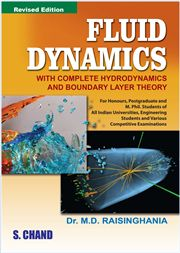# FLUID DYNAMICS: WITH COMPLETE HYDRODYNAMICS AND BOUNDARY LAYER THEORY, 12/e DR. M.D. RAISINGHANIA-S.CHAND

525.00 475.00

Introduction • Kinematics of Fluids in Motion • Equations of Motion of Inviscid Fluids • One-Dimensional Inviscid Incompressible Flow (Bernoulli's Equation and its Applications) • Motion in Two-Dimensions Sources and Sinks • General Theory of Irrotational Motion • Motion of Cylinders • The Use of Conformal Representation Aerofoils • Discontinuous Motion • Irrotational Motion in Three-Dimensions. Motion of a Sphere Stokes's Stream Function • Vortex Motion. Rectilinear Vortices • Waves • Generaltheory of Stress and Rate of Strain • The Navier-Stokes Equations and the Energy Equation • Dynamical Similarity, Inspection Analysis and Dimensional Analysis • Laminar Flow of Viscous Incompressible Fluids • Theory of Very Slow Motion • Boundary Layer Theory • Thermal Boundary Layer • Flow of Inviscid Compressible Fluids. Gas Dynamics • Flow of a Compressible Viscous Fluid